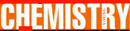# Schrödinger Equation - Research Article from World of Chemistry

This encyclopedia article consists of approximately 3 pages of information about Schr&ouml;dinger Equation.
 This section contains 714 words (approx. 3 pages at 300 words per page) View a FREE sampleIn 1924, Louis-Victor de Broglie showed that any particle, such as an electron, has wave-like properties. Electrons up to that point were treated as hard particles, much like billiard balls. De Broglie's theory changed this view though, and in 1926, Erwin Schröodinger formulated a wave equation that treated an electron as a wave, rather than as a particle.

A wave is an any oscillating disturbance that moves through space, or a medium like air or water. To a physicist, a wave of a quantum entity is essentially the same as a wave on a lake. Common waves, like those on lakes, are described by mathematical equations, called wave equations. If common everyday waves can be described by equations, then an electron's wave should also be able to be described by a mathematical wave equation. Schrödinger was the first to mathematically...

(read more)

 This section contains 714 words (approx. 3 pages at 300 words per page) View a FREE sampleMore summaries and resources for teaching or studying Schrdinger Equation.
CopyrightsSchrödinger Equation from Gale. ©2005-2006 Thomson Gale, a part of the Thomson Corporation. All rights reserved.